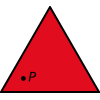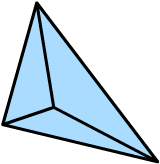#### You may also like### Fitting In

The largest square which fits into a circle is ABCD and EFGH is a square with G and H on the line CD and E and F on the circumference of the circle. Show that AB = 5EF. Similarly the largest equilateral triangle which fits into a circle is LMN and PQR is an equilateral triangle with P and Q on the line LM and R on the circumference of the circle. Show that LM = 3PQ### Triangle Midpoints

You are only given the three midpoints of the sides of a triangle. How can you construct the original triangle?### Three Way Split

Take any point P inside an equilateral triangle. Draw PA, PB and PC from P perpendicular to the sides of the triangle where A, B and C are points on the sides. Prove that PA + PB + PC is a constant.

# Tetrahedra Tester

##### Age 14 to 16Challenge Level

An irregular tetrahedron is composed of four different triangles.

Can such a tetrahedron be constructed where the side lengths are 4, 5, 6, 7, 8 and 9 units of length?

How many different tetrahedra can be constructed?

Explain how you know you have found all the possible tetrahedra.